# 1 Introduction

Biomarkers are widely used in pharmaceutical industry for drug discovery and development at various stages, from preclinical animal study to phase I- III and post market clinical trials, and can be used for target identification, diseased diagnostics, patient stratification, treatment prediction and etc. High-throughput assay technology enables the collection of various types of biomarkers, such as gene expression and various omics biomarkers. In high-throughput assays, a large number of biomarkers are measured in a single experiment, but subject to substantial known or unknown variability. Hence biomarkers from high-throughput assays yield two major characteristics, including high dimensionality of the data and relative large data variability. Due to the two characteristics, it is critical and challenging to visualize the biomarker data and corresponding statistical results to ensure the data quality and the reliability of downstream statistical analysis results. Therefore we developed this R package as a visualization tool for generating the commonly used plots in analyzing biomarkers from high-throughput assays, including data quality control, individual biomarker analysis and multivariate analysis. The tool also included an analysis pipeline for analyzing biomarkers in the setting of two groups comparison with the flexibility to extend to customized or project specific analysis.

The R package statVisual provide novel solutions to the users by utilizing many powerful R base functions and R packages. For example, the function hist in the R package graphics can draw the histogram for a set of observations. However, to visualize histograms for two or more groups of observations in one figure, the users need to write their own code. The R package statVisual provides the function Hist to help the users to obtain such figure in one command. This vignette illustrates the usages of the functions provided by the R package statVisual.

# 2 System Requirements

R (>=3.5.0) is required to run the R package statVisual properly.

The following R packages are required to be installed before installing and running statVisual:

# packages in Bioconductor
library(Biobase)    # base package for Bioconductor
library(limma)      # linear models for continuous omics data
library(pvca)       # principal variance component analysis

# packages in CRAN
library(dplyr)      # data manipulation and pipe operation
library(factoextra) # extract and visualize results of multivariate data analysis
library(forestplot) # forest plot
library(gbm)        # generalized boosted regression models
library(GGally)     # extension to 'ggplot2'
library(ggdendro)   # dendrogram for data clustering
library(ggfortify)  # data visualization tools for statistical analysis results
library(ggplot2)    # create graphics based on "The Grammer of Graphics"
library(ggrepel)    # tidy text display in ggplot
library(glmnet)     # cross validation plot for glmnet
library(grDevices)  # R graphics devices and support for colors and fonts
library(gridExtra)  # Grid graphics
library(knitr)      # dynamic report generation
library(methods)    # formal methods and classes
library(pROC)       # display and analyze ROC curves
library(randomForest) # Random forest variable importance
library(reshape2)   # flexibly reshape data
library(rmarkdown)  # dynamic documents for R
library(rpart.plot) # plots for recursive partitioning for classification, regression and survival trees
library(tibble)     # simple data frames
library(stats)      # basic statistical functions

library(statVisual)

To check the information about the statVisual package, please type the following R statement:

library(help = statVisual)

To find the usage of a function (e.g., Hist) in statVisual, please use the help function or use ?. For example,

help(Hist)
?Hist

# 4 Available functions and datasets

## 4.1 Available functions

Below is a list of currently available functions in statVisual for plotting.

• Analysis focusing on one outcome variable:
• Hist - Compare groups based on histograms
• Den - Compare groups based on density plots
• Analysis focusing on two outcome variables:
• XYscatter - Compare groups based on scatter plots
• stackedBarPlot - Compare groups based on bar plots
• BiAxisErrBar - Compare groups based on bi-axis error bar plots
• Analysis of longitudinal data:
• LinePlot - Compare groups based on trajectory plots (commonly used to visualize tumor volume data)
• Box - Compare groups based on boxplots across time
• ErrBar - Compare groups based on dotplots across time
• barPlot - Compare groups based on barplots across time
• Analysis focusing on pattern detection:
• Dendro - Compare groups based on dendrogram of hierarchical clustering
• iprcomp - Calculate principal components (missing values allowed)
• PCA_score - Scatter plot of the 2 specified PCs
• Heat - Heatmap with row names colored by groups
• PVCA - PVCA plot
• Volcano - Volcano Plot with option to label significant results
• Analysis focusing on prediction:
• BoxROC - Compare boxplots with ROC curve
• cv_glmnet_plot - Cross validation plot of glmnet
• ImpPlot - Plot of variable importance
• The overall wrapper function:
• statVisual - the wrapper function

## 4.2 Available datasets

• diffCorDat: A simulated dataset for illustrating differential correlations between cases and controls

The simulated data set diffCorDat contains expression levels of 2 gene probes for 50 cases and 50 controls. The expression levels of probe1 are generated from $$N(0, 1)$$. The expression levels of probe2 for controls are also generated from $$N(0, 1)$$. The expression levels of probe 2 for cases are generated from the formula $\begin{equation} probe2_{i} = -probe1_{i} + e_i, i=1, \ldots, nCases, \end{equation}$ where $$e_i\sim N(0, 0.3^2)$$.

That is, the expression levels of probe 1 and probe 2 are negatively correlated in cases, but not correlated in controls.

To load diffCorDat, we can use the following R code:

data(diffCorDat)

print(dim(diffCorDat))
#>  100   3
print(diffCorDat[1:2,])
#>      probe1     probe2   grp
#> 1 0.1567038  0.2438042 cases
#> 2 1.3738112 -1.5249113 cases
• esSim: A simulated gene expression dataset for differential expression analysis

The dataset esSim was generated based on the R code in the manual of the function of the R Bioconductor package . There are 100 probes and 20 samples (10 controls and 10 cases). The first 3 probes are over-expressed in cases. The 4-th and 5-th probes are under-expressed in cases. The remaining 95 probes are non-differentially expressed between cases and controls. Expression levels for 100 probes were first generated from normal distribution with mean 0 and standard deviation varying between probes ($$sd=0.3\sqrt{4/\chi^2_4}$$). For the 3 OE probes, we add 2 to the expression levels of the 10 cases. For the 2 UE probes, we subtract 2 from the expression levels of the 10 cases.

To load esSim, we can use the following R code:

data(esSim)

print(dim(esSim))
#> Features  Samples
#>      100       20
print(esSim)
#> ExpressionSet (storageMode: lockedEnvironment)
#> assayData: 100 features, 20 samples
#>   element names: exprs
#> protocolData: none
#> phenoData
#>   sampleNames: subj1 subj2 ... subj20 (20 total)
#>   varLabels: sid grp
#> featureData
#>   featureNames: probe1 probe2 ... probe100 (100 total)
#>   fvarLabels: probeid gene chr
#> experimentData: use 'experimentData(object)'
#> Annotation:
• genoSim: A simulated genotype dataset

genoSim is an ExpressionSet object containing genotype data of 10 SNPs for 100 subjects (50 cases and 50 controls). Eight of SNPs have same minor allele frequency ($$MAF=0.2$$) between cases and controls. The other 2 SNPs have the different MAFs between cases and controls ($$MAF_{cases}=0.4$$ and $$MAF_{controls}=0.2$$).

We assume Hardy-Weinberg Equilibrium. That is, the genotype for wild-type homozygote is $$(1-MAF)^2$$; the genotype for heterozygote is $$2*MAF*(1-MAF)$$; and the genotype for mutant homozygote is $$MAF^2$$.

The phenotype of the ExpressionSet object contains two variables: subject id ($$sid$$) and case-control status ($$grp$$).

The feature data contains two variables: snp id ($$snp$$) and SNP significance status ($$memSNPs$$).

• longDat: A simulated dataset for longitudinal data analysis

The dataset longDat is generated from the following mixed effects model for repeated measures: $\begin{equation} y_{ij}=\beta_{0i}+\beta_1 t_{j} + \beta_2 grp_{2i} + \beta_3 grp_{3i} + \beta_4 \times\left(t_{j}\times grp_{2i}\right) + \beta_5 \times\left(t_{j}\times grp_{3i}\right) +\epsilon_{ij}, \end{equation}$ where $$y_{ij}$$ is the outcome value for the $$i$$-th subject measured at $$j$$-th time point $$t_{j}$$, $$grp_{2i}$$ is a dummy variable indicating if the $$i$$-th subject is from group 2, $$grp_{3i}$$ is a dummy variable indicating if the $$i$$-th subject is from group 3, $$\beta_{0i}\sim N\left(\beta_0, \sigma_b^2\right)$$, $$\epsilon_{ij}\sim N\left(0, \sigma_e^2\right)$$, $$i=1,\ldots, n, j=1, \ldots, m$$, $$n$$ is the number of subjects, and $$m$$ is the number of time points.

When $$t_j=0$$, the expected outcome value is $\begin{equation} E\left(y_{ij}\right)=\beta_0+\beta_2 dose_{2i} + \beta_3 dose_{3i}. \end{equation}$ Hence, we have at baseline $\begin{equation} E\left(y_{ij}\right)=\beta_0,\; \mbox{for dose 1 group},\\ E\left(y_{ij}\right)=\beta_0 + \beta_2,\; \mbox{for dose 2 group},\\ E\left(y_{ij}\right)=\beta_0 + \beta_3,\; \mbox{for dose 3 group}. \end{equation}$

For dose 1 group, the expected outcome values across time is $\begin{equation} E\left(y_{ij}\right)=\beta_0+\beta_1 t_{j}. \end{equation}$

We also can get the expected difference of outcome values between dose 2 group and dose 1 group, between dose 3 group and dose 1 group, and between dose 3 group and dose 2 group: $\begin{equation} E\left(y_{ij} - y_{i'j}\right) =\beta_2+\beta_4 t_{j},\;\mbox{for subject i in dose 2 group and subject i' in dose 1 group}, \end{equation}$

$\begin{equation} E\left(y_{kj} - y_{i'j}\right) =\beta_3+\beta_5 t_{j},\;\mbox{for subject k in dose 3 group and subject i' in dose 1 group}, \end{equation}$

$\begin{equation} E\left(y_{kj} - y_{ij}\right) =\left(\beta_3 -\beta_2\right)+\left(\beta_5-\beta_4\right) t_{j},\;\mbox{for subject k in dose 3 group and subject i in dose 2 group}. \end{equation}$

We set $$n=90$$, $$m=6$$, $$\beta_0=5$$, $$\beta_1=0$$, $$\beta_2=0$$, $$\beta_3=0$$, $$\beta_4=2$$, $$\beta_5=-2$$, $$\sigma_e=1$$, $$\sigma_b=0.5$$, and $$t_{j}=j$$, $$j=0, \ldots, m-1$$.

That is, the trajectories for dose 1 group are horizontal with mean intercept at $$5$$, the trajectories for dose 2 group are linearly increasing with slope $$2$$ and mean intercept $$5$$, and the trajectories for dose 3 group are linearly decreasing with slope $$-2$$ and mean intercept $$5$$.

To load longDat, we can use the following R code:

data(longDat)

print(dim(longDat))
#>  540   4
print(longDat[1:2,])
#>   sid  time        y  grp
#> 1   1 time0 4.539033 grp1
#> 2   1 time1 5.738715 grp1

# 5 Analysis focusing on one outcome variable:

## 5.1 Compare Groups Based on Histograms

A common task in statistical comparison is to compare the mean values among groups. The reason to comparing the summary statistics (means) is to simplify the problem of comparing two distributions since it is hard to numerically compare two distributions. However, we can easily compare two distributions by visualizing the empirical distributions (e.g., histograms).

To compare histograms across groups, we can use the function Hist:

# expression data
dat = exprs(esSim)
print(dim(dat))
#>  100  20
print(dat[1:2,])
#>           subj1    subj2    subj3    subj4    subj5    subj6    subj7    subj8
#> probe1 1.462929 1.596203 2.252267 1.974616 1.842606 1.677204 2.242665 1.705923
#> probe2 1.574342 2.232537 1.846260 1.980999 2.483777 2.048599 1.786081 1.934728
#>           subj9   subj10     subj11     subj12      subj13    subj14     subj15
#> probe1 1.758028 1.919597 0.07477602  0.3054811  0.12356526 0.3221119 -0.1346480
#> probe2 1.740329 1.942189 0.08308643 -0.1012774 -0.06669481 0.5413783  0.0245575
#>             subj16     subj17    subj18     subj19     subj20
#> probe1 -0.03845011  0.3504460 0.4173905 -0.1438801  0.3335083
#> probe2 -0.03849619 -0.2536194 0.1420066 -0.1072697 -0.1750033

# phenotype data
pDat = pData(esSim)
print(dim(pDat))
#>  20  2
print(pDat[1:2,])
#>         sid grp
#> subj1 subj1   1
#> subj2 subj2   1

# feature data
fDat = fData(esSim)
print(dim(fDat))
#>  100   3
print(fDat[1:2,])
#>        probeid      gene chr
#> probe1  probe1 fakeGene1   1
#> probe2  probe2 fakeGene2   1

# choose the first probe which is over-expressed in cases
pDat$probe1 = dat[1,] # check histograms of probe 1 expression in cases and controls pDat$grp=factor(pDat$grp) print(table(pDat$grp, useNA = "ifany"))
#>
#>  0  1
#> 10 10
Hist(
data = pDat,
y = 'probe1',
group = 'grp')


We also can use the wrapper function statVisual:

statVisual(type = 'Hist',
data = pDat,
y = 'probe1',
group = 'grp')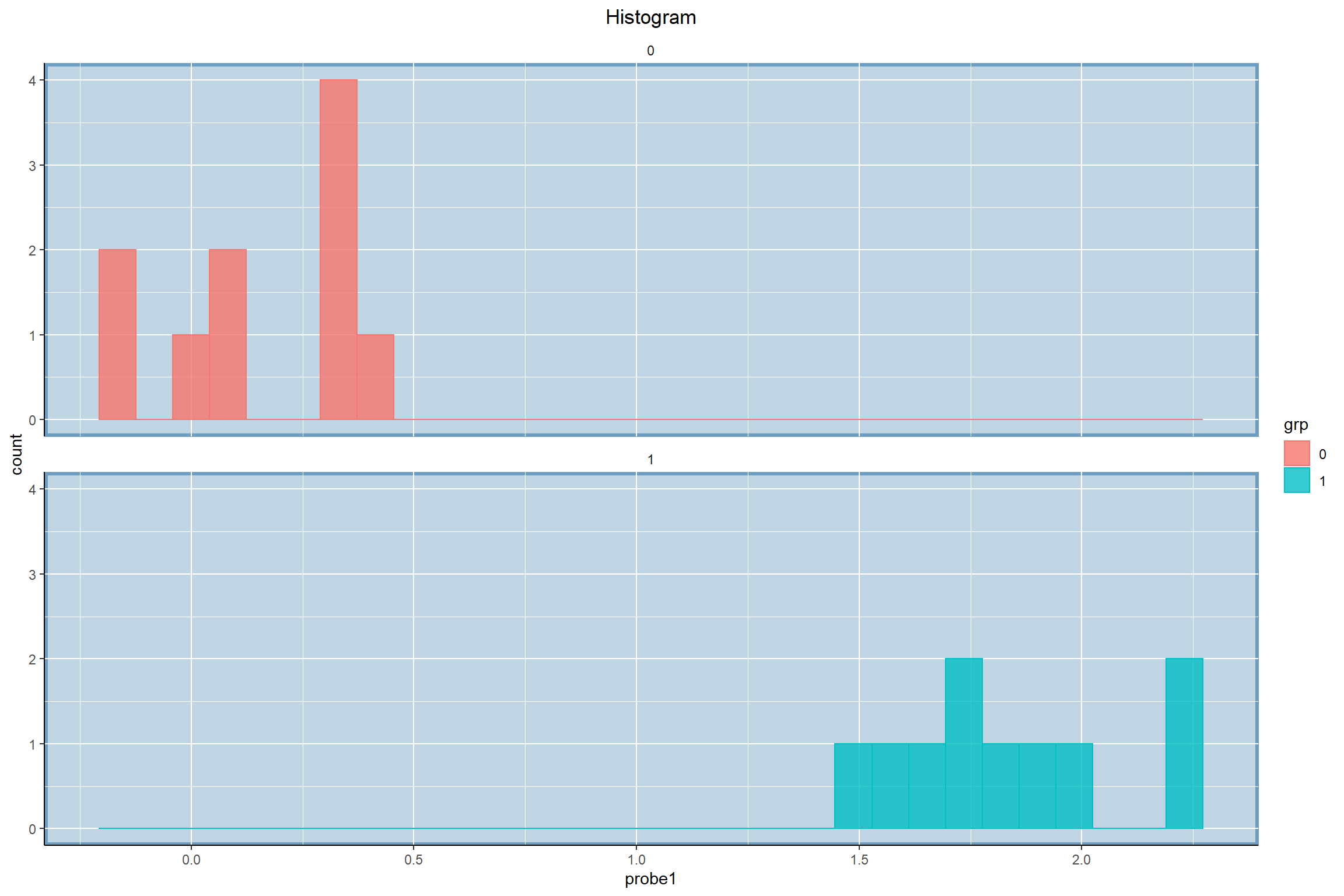## 5.2 Compare Groups Based on Density Plots

We can compare two distribution by comparing the estimated density functions.

The function Den is used to visualize the differences of densities across groups.


Den(
data = pDat,
y = 'probe1',
group = 'grp') 

We can also use the wrapper function statVisual:

statVisual(type = 'Den',
data = pDat,
y = 'probe1',
group = 'grp')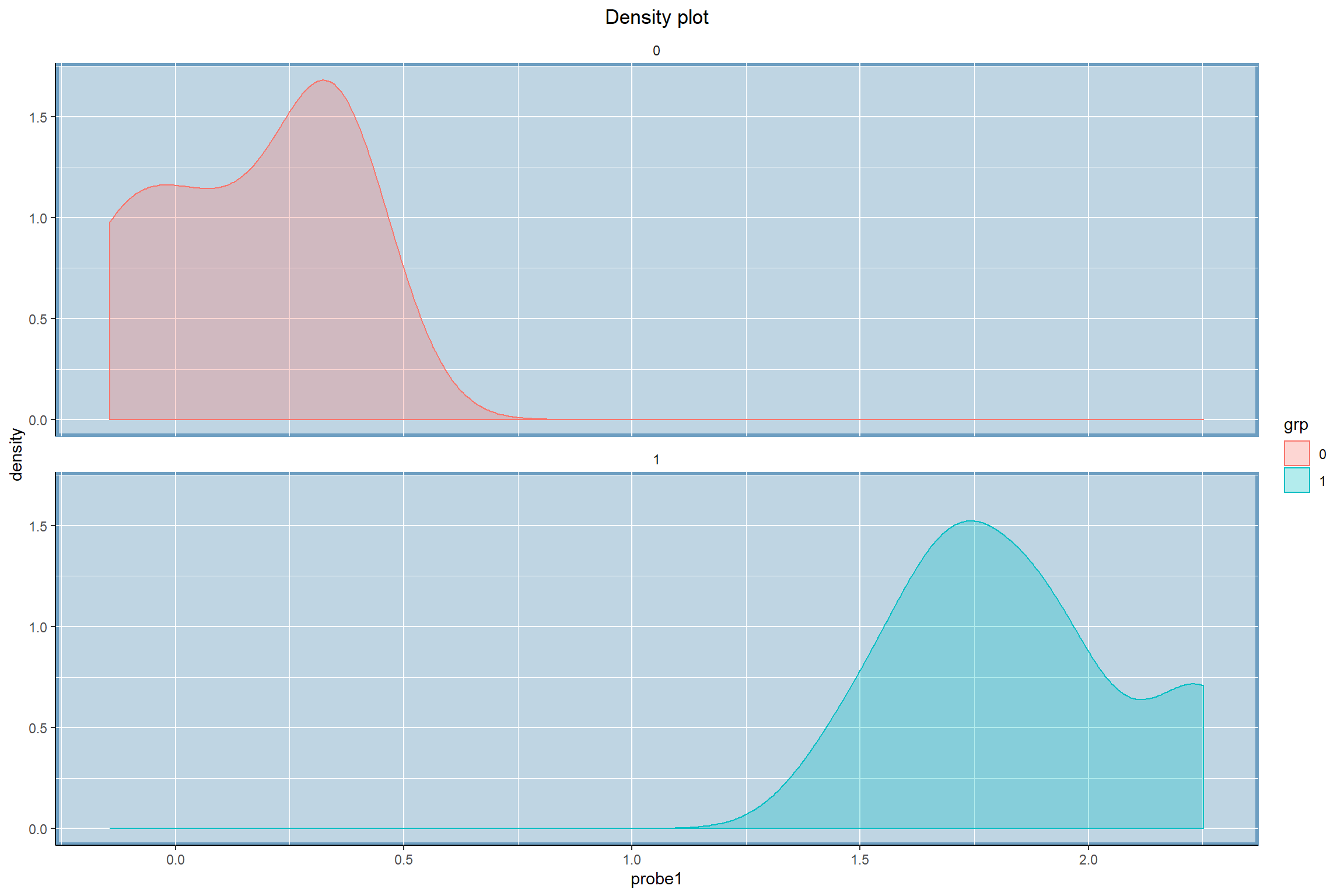# 6 Analysis focusing on two outcome variables:

## 6.1 Compare Groups Based on Scatter Plots

The correlation is an important statistic to evaluate the linear relationship between two continuous variables. To check the linearity and to visualize the strength of the linear relationship, we can draw scatter plot. Some time, it is of interest to compare the correlations among groups. The function XYscatter can help the comparison by display the scatter plots across groups in one figure:

For example, to check if the relationship between Sepal length vs. Sepal width is the same across different species, we can use the R code:

XYscatter(
data = diffCorDat,
x = 'probe1',
y = 'probe2',
group = 'grp',
title = 'Scatter Plot: probe1 vs probe2')


We can also use the wrapper function statVisual:

statVisual(type = 'XYscatter',
data = diffCorDat,
x = 'probe1',
y = 'probe2',
group = 'grp',
title = 'Scatter Plot: probe1 vs probe2')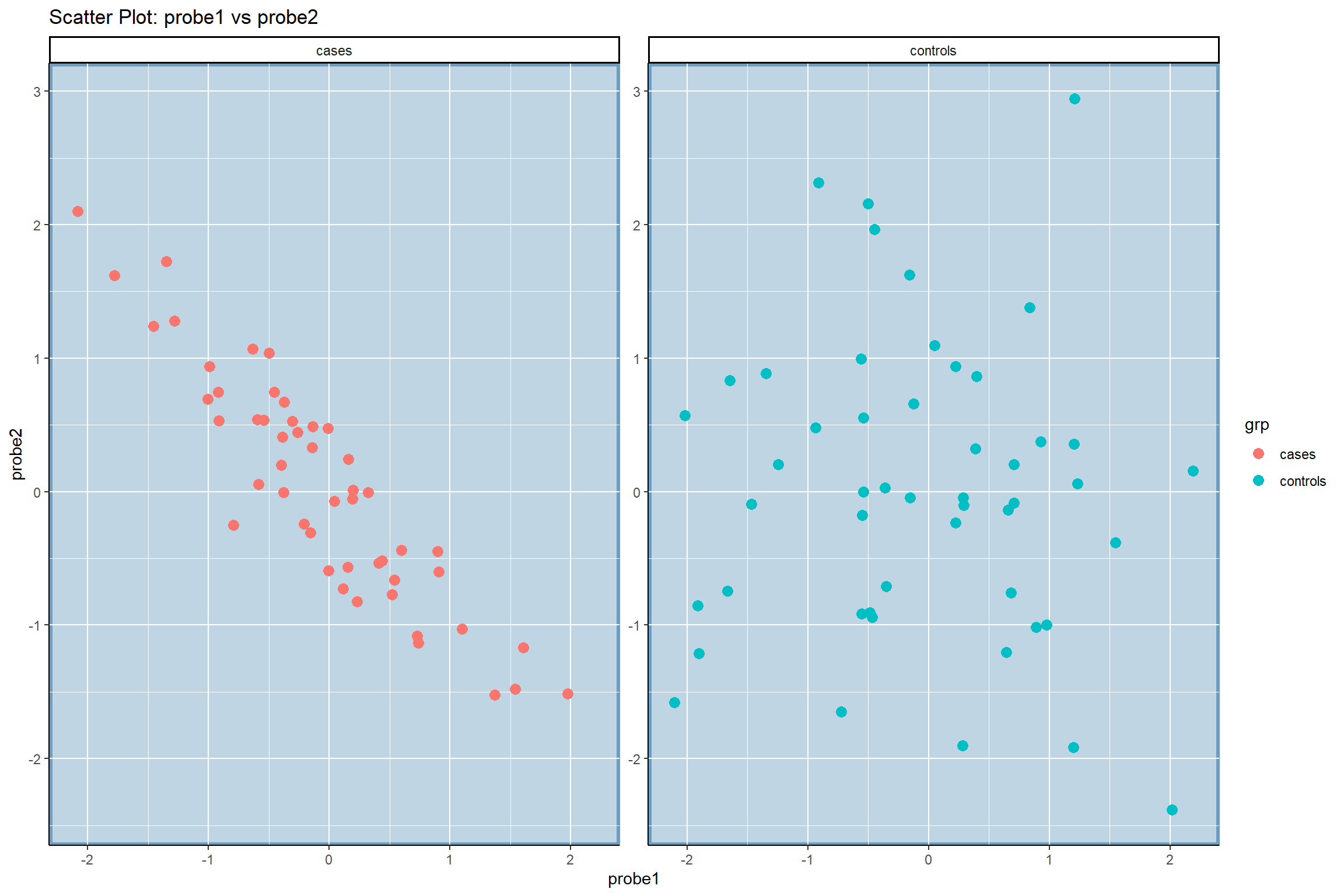## 6.2 Compare Groups Based on Bar Plots

For two categorical variables, we can use the function stackedBarPlot to show their association.

For example, in the ExpressionSet object genoSim, there are simulated genotypes of 10 SNPs for 50 cases and 50 control. If we would like to know if the pattern of the genotypes of the SNP 1 in cases is the same as that in controls, we can draw bar plots.

data(genoSim)

pDat = pData(genoSim)
geno = exprs(genoSim)

pDat$snp1 = geno[1,] print(table(pDat$snp1, pDat$grp, useNA="ifany")) #> #> 0 1 #> 0 37 19 #> 1 11 22 #> 2 2 9 stackedBarPlot(dat = pDat, catVar = "snp1", group = "grp", xlab = "snp1", ylab = "Count", group.lab = "grp", title = "Stacked barplots of counts for SNP1", catVarLevel = NULL) We can also use the wrapper function statVisual: statVisual(type = 'stackedBarPlot', dat = pDat, catVar = "snp1", group = "grp", xlab = "snp1", ylab = "Count", group.lab = "grp", title = "Stacked barplots of counts for SNP1", catVarLevel = NULL)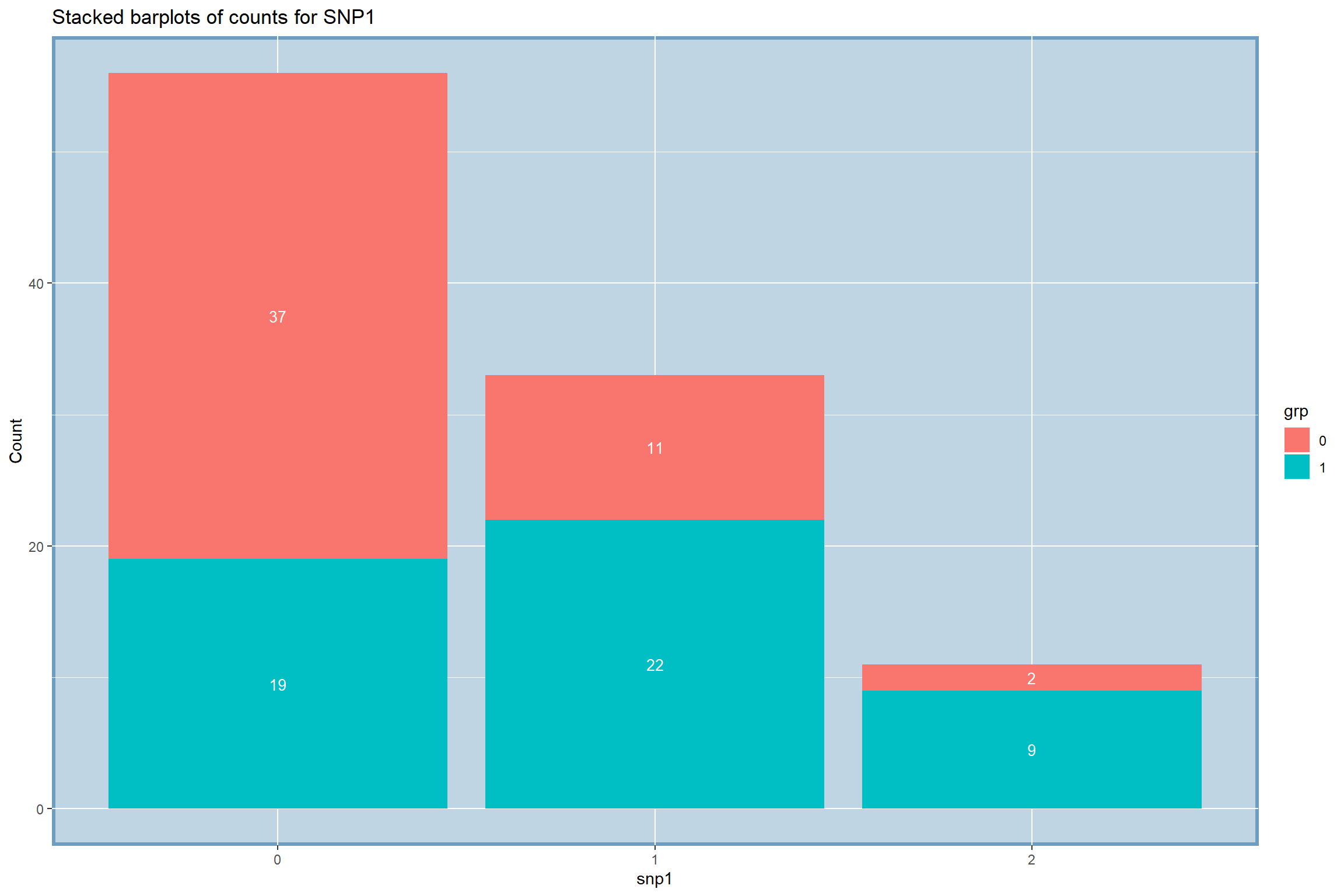Note that the input parameter catVarLevel can be used to change the order of the values of catVar shown in x-axis. For example, statVisual(type = 'stackedBarPlot', dat = pDat, catVar = "snp1", group = "grp", xlab = "snp1", ylab = "Count", group.lab = "grp", title = "Stacked barplots of counts for SNP1", catVarLevel = c(2, 0, 1))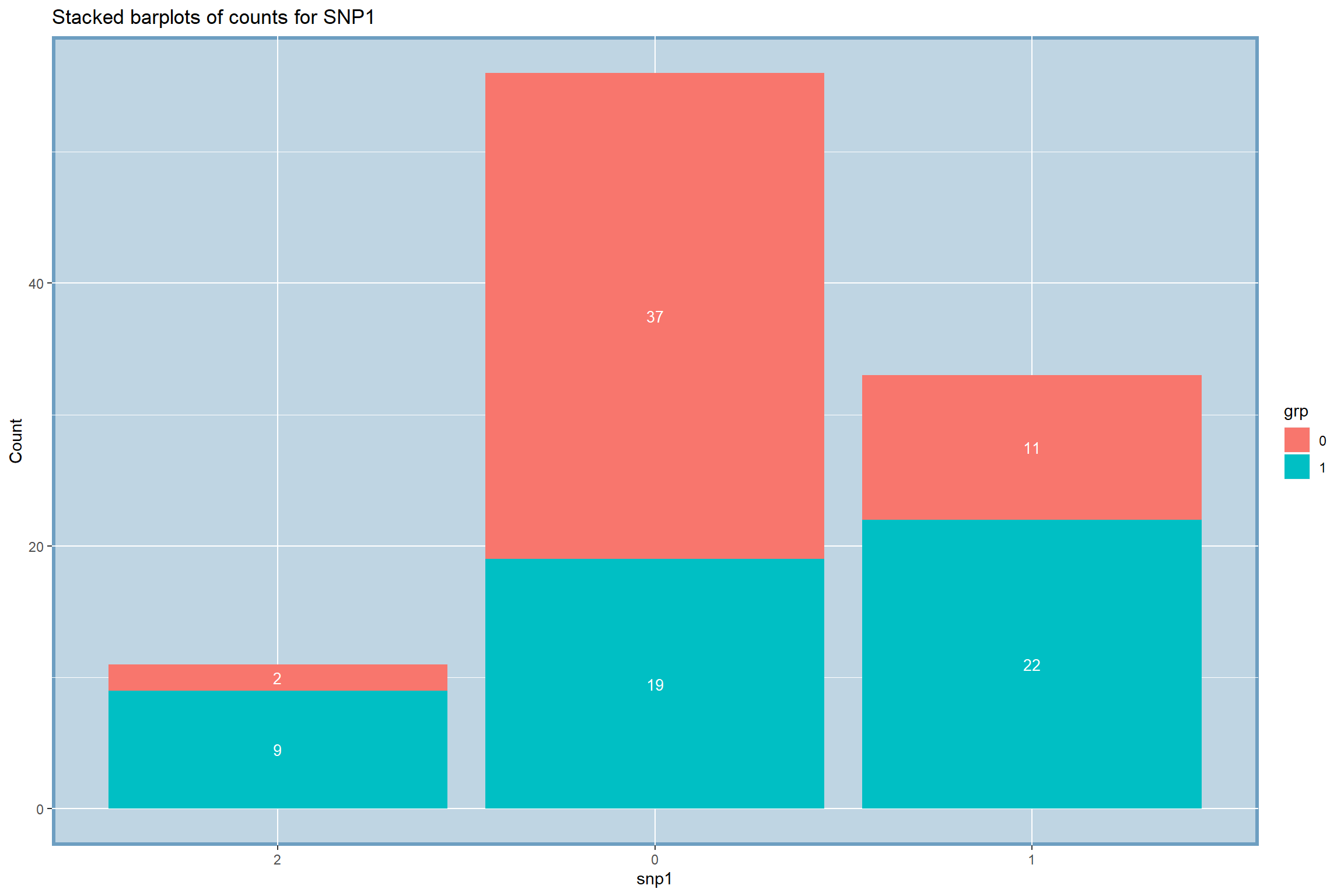## 6.3 Compare Groups Based on Bi-Axis Error Bar Plots Some time, we would like to compare two outcomes with different scales across groups in one figure using error bar plots. The function BiAxisErrBar can do this task. Each bar plot displays mean standard error.  library(tidyverse) library(ggplot2) print(head(mtcars)) #> mpg cyl disp hp drat wt qsec vs am gear carb #> Mazda RX4 21.0 6 160 110 3.90 2.620 16.46 0 1 4 4 #> Mazda RX4 Wag 21.0 6 160 110 3.90 2.875 17.02 0 1 4 4 #> Datsun 710 22.8 4 108 93 3.85 2.320 18.61 1 1 4 1 #> Hornet 4 Drive 21.4 6 258 110 3.08 3.215 19.44 1 0 3 1 #> Hornet Sportabout 18.7 8 360 175 3.15 3.440 17.02 0 0 3 2 #> Valiant 18.1 6 225 105 2.76 3.460 20.22 1 0 3 1 print(table(mtcars$gear, useNA="ifany"))
#>
#>  3  4  5
#> 15 12  5

BiAxisErrBar(
dat = mtcars,
group = "gear",
y.left = "mpg",
y.right = "wt")


We can also use the wrapper function statVisual:

statVisual(type = "BiAxisErrBar",
dat= mtcars,
group = "gear",
y.left = "mpg",
y.right = "wt")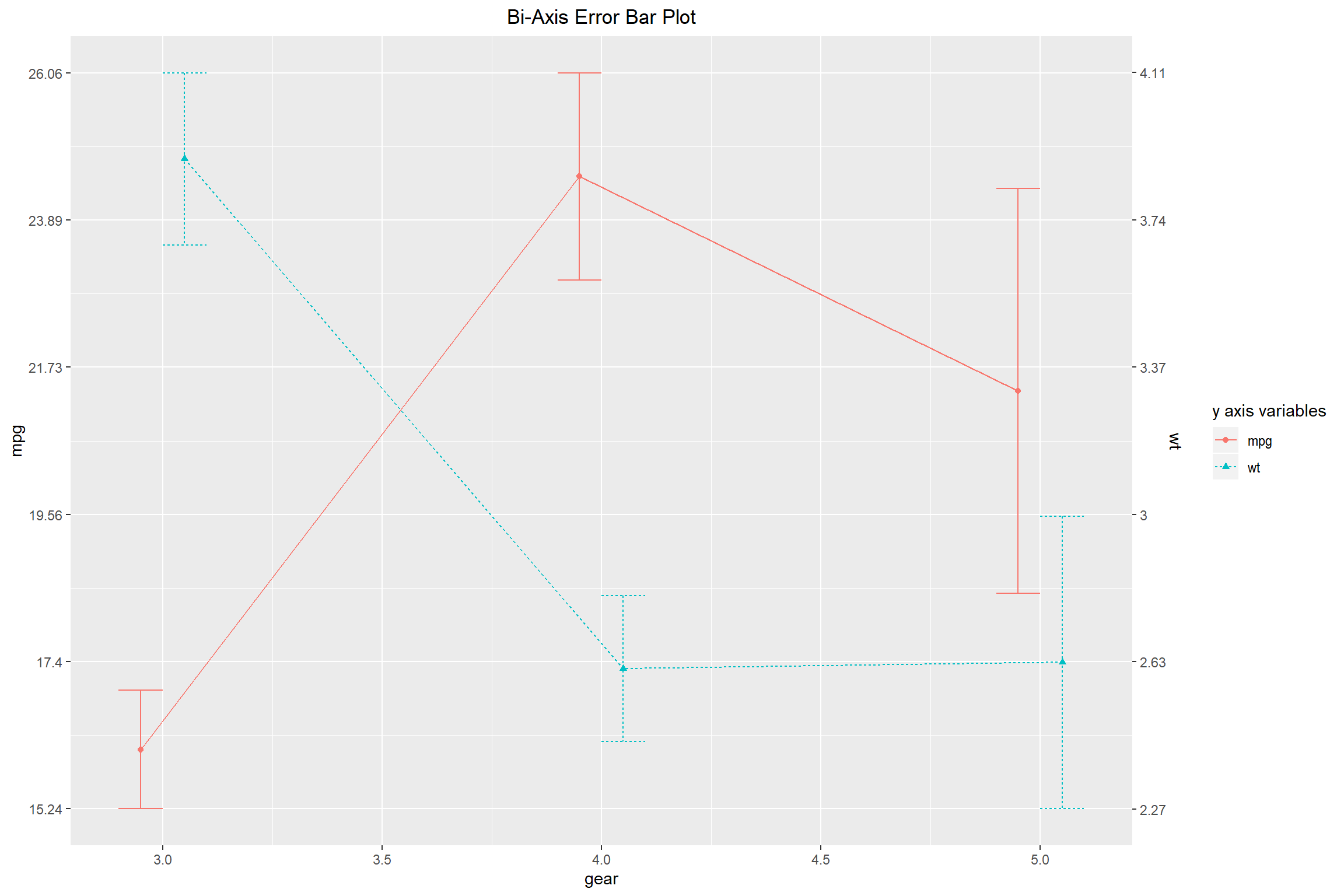# 7 Analysis of Longitudinal Data

## 7.1 Compare Groups Based on Trajectory Plots

In clinical trial, it is common to collect data at multiple time points. Therefore, it is natural to compare groups based on the trajectories of individual subjects across time. The function LinePlot can do this task:


LinePlot(
data = longDat,
x = 'time',
y = 'y',
sid = 'sid',
group = 'grp')


We also can use the wrapper function statVisual:

statVisual(type = "LinePlot",
data = longDat,
x = 'time',
y = 'y',
sid = 'sid',
group = 'grp')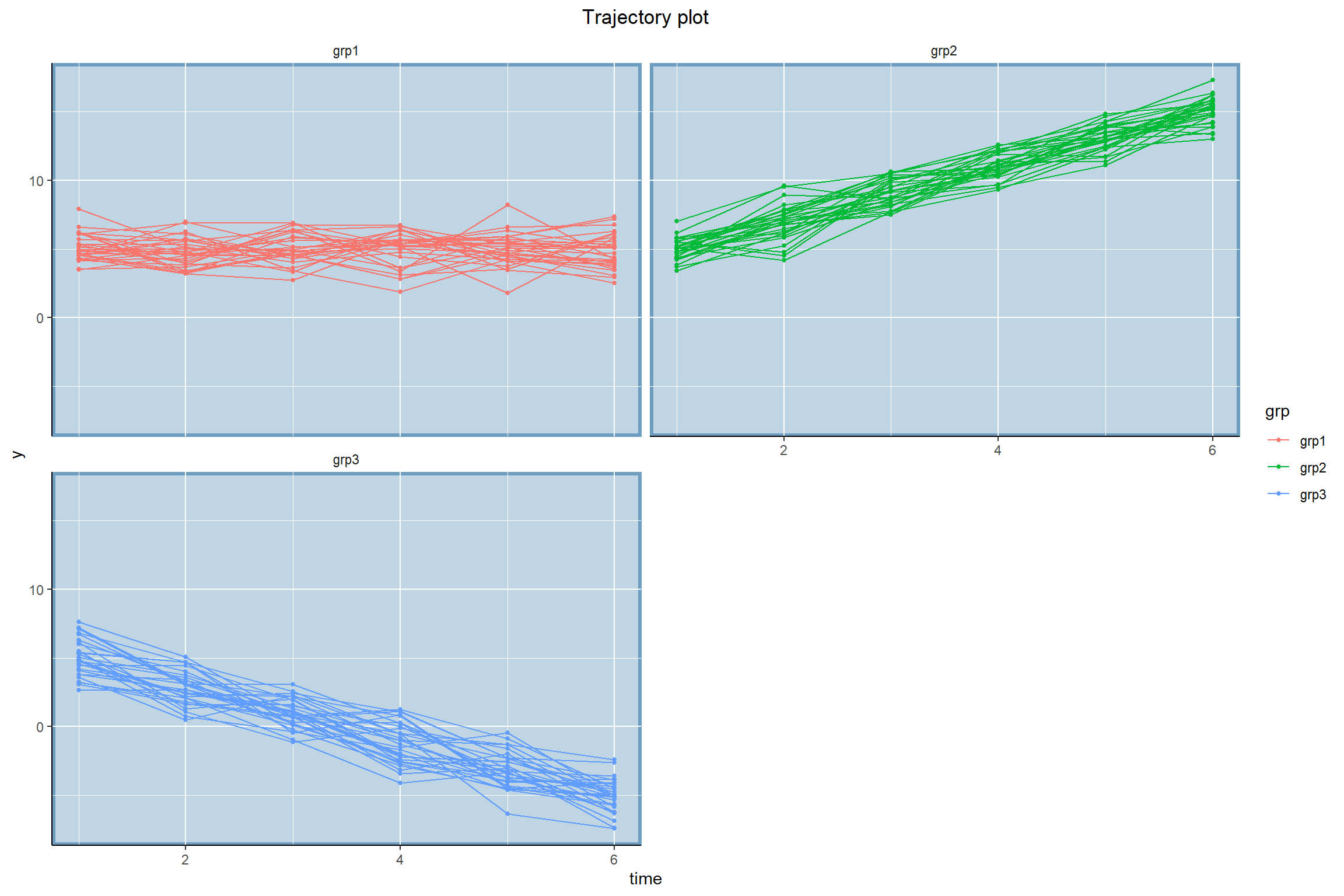## 7.2 Compare Groups Based on Box Plots Across time

If there are many individuals in a longitudinal dataset, the trajectory plot might look messy. In this case, we develop the function Box to compare groups using boxplots at each time point. In addition, line segments are used to connect the mean/median of each boxplot of the same group across time to show the differences between the mean trajectories. Note that this function is suitable for the scenarios where observations of all subjects are measured at a few fixed time points.

library(dplyr)
Box(
data = longDat,
x = 'time',
y = 'y',
group = 'grp',
title = "Boxplots across time") 

Or we can use the wrapper function statVisual:

statVisual(type = 'Box',
data = longDat,
x = 'time',
y = 'y',
group = 'grp',
title = "Boxplots across time")